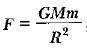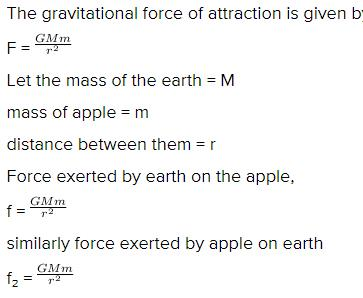Courses

# Important Questions (1 mark): Gravitation

## 20 Questions MCQ Test Science Class 9 | Important Questions (1 mark): Gravitation

Description
This mock test of Important Questions (1 mark): Gravitation for Class 9 helps you for every Class 9 entrance exam. This contains 20 Multiple Choice Questions for Class 9 Important Questions (1 mark): Gravitation (mcq) to study with solutions a complete question bank. The solved questions answers in this Important Questions (1 mark): Gravitation quiz give you a good mix of easy questions and tough questions. Class 9 students definitely take this Important Questions (1 mark): Gravitation exercise for a better result in the exam. You can find other Important Questions (1 mark): Gravitation extra questions, long questions & short questions for Class 9 on EduRev as well by searching above.
QUESTION: 1

Solution:
QUESTION: 2

Solution:
QUESTION: 3

### The constant G

Solution:

The small size of the gravitational constant G  tells you that  the gravitational force is actually quite weak compared to other known  forces  like the electric, magnetic and  nuclear forces.

QUESTION: 4

Acceleration due to gravity is

Solution:
QUESTION: 5

If the radius of earth were to shrink by 1%, its mass remaining the same, the acceleration due to gravity on earth’s surface would

Solution:
QUESTION: 6

An apple and a stone dropped from certain height accelerates

Solution:
QUESTION: 7

The gravitational force between two bodies does not depend on

Solution:
QUESTION: 8

Weight of 1 kg is

Solution:
QUESTION: 9

10 kg wt equals

Solution:
QUESTION: 10

A body at rest having uniform acceleration is

Solution:

The correct option is Option C.

A body at rest having uniform acceleration is any body thrown vertically up against gravity.

When a body is thrown vertically upwards, at the highest point only velocity is zero because the acceleration due to gravitational force is acting downward continuously and that is the reason for velocity being zero at the highest point, hence velocity is zero because of the acceleration.

QUESTION: 11

At which of the following locations is the value of g largest?

Solution:
QUESTION: 12

acceleration due to gravity varies with

Solution:
QUESTION: 13

Two objects of different masses falling freely near the surface of moon would

Solution:

When an object is under free fall, its acceleration depends only on the acceleration due to gravity and mass has no role to play. Due to this; irrespective of their masses, the object under free fall with have the same velocity at any instant.

QUESTION: 14

Two particles are placed at some distance. If the mass of each of the two particles is doubled, keeping the distance between them unchanged, the value of gravitational force between them will be

Solution:
QUESTION: 15

In the relation, the quantity  G

Solution:
QUESTION: 16

The force of attraction between two unit point masses separated by a unit distance is called

Solution:
QUESTION: 17

Two particles are placed at some distance. If mass of each of the two particles is doubled, keeping the distance between them unchanged, the value of gravitational force between them will become/remain

Solution:
QUESTION: 18

An apple falls from a tree because of gravitational attraction between the earth and apple. If F1 is the magnitude of force exerted by the earth on the apple and F2 is the magnitude of force exerted by apple on earth, then

Solution:so we can see that f is equal to f₂

QUESTION: 19

The weight of an object at the centre of the earth of radius R is

Solution:

The weight of the body at the surface of the earth is equal to zero. The accelertion due to gravity is maximum on the surface of the earth and as we towards the centre of the equator it becomes zero.

The acceleration due to gravity varies due to the shape of the earth. The radius of the earth is more at the equator than at the poles.

Thus, the value of g at the centre of the earth is zero.

QUESTION: 20

Freely falling objects weight ._______.

Solution:

An object kept in a lift which falls freely  , weighs zero on the weighing machine , but its actual  weight is still mg. This happens  because the normal reaction force Exerted on the object in the lift is equal to mg,which in turn equals the  weight of the  object.